#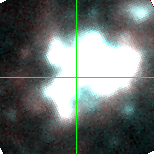M33C-21057 (J013433.10+304659.0)

## Target Notes### Classification: HII

Target is embedded in the heart of massive HII region NGC 604. The target is unresolved from 6 LGGS stars >2 magnitudes fainter within 2 arcseconds. 12 other LGGS stars within 5 arcseconds are not picked out or properly modeled. Measures are marked "B" by default and "X" if there are problems with the PSF fit.

## Plots of Brightness and Color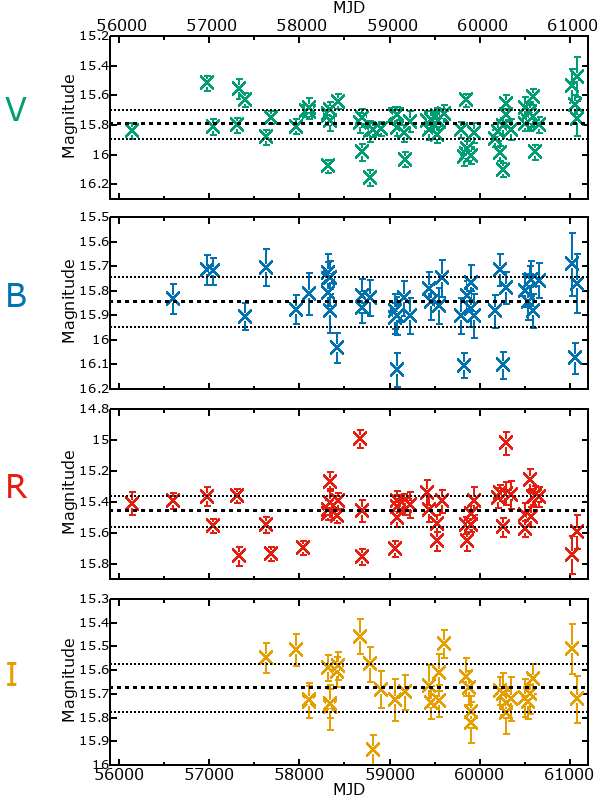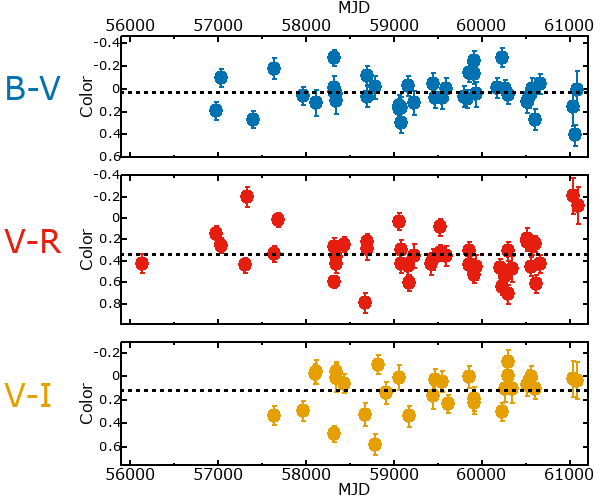## Variability

From the data available in this survey, it is not possible to tell with certainty if this star is variable.

### Correlation factors between bands

If the brightness variation is true variability then there should be a correlation between brightness changes recorded in different filter bands. The following table are the R-squared linear correlation factors calculated for the pairs of bands. The number in parentheses next to the R-squared value is the number of elements used to calculate the correlation factor. The number in square brackets next to the band is the range of magnitudes measured.

V [0.35 mag]B [0.63 mag]R [0.82 mag]
B [0.35 mag]0.05 (26)
R [0.82 mag]0.07 (26)0.17 (20)
I [0.36 mag]0.51 (15)0.06 (9)0.28 (12)

### Magnitude-Magnitude Plots

The plots below are provided to check the values in the table above and also to show any non-linear correlations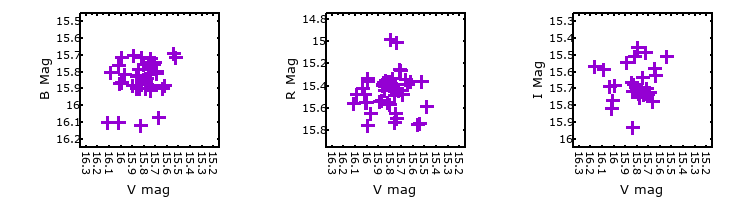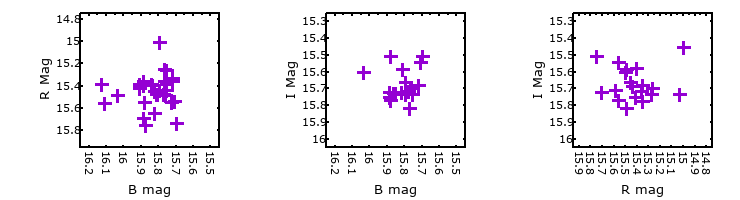### I & R Statistics

Welch & Stetson (1993, AJ, 105, 1813) present a method for varible star detection in CCD images from automated surveys. Data from two bands are compared and two statistics are calculated: R and I.

The I-statistic has an expectation value of zero for stars that are NOT variable. A larger non-zero I-value indicates a higher likelihood that the star is variable. The R statistic indicates how the color of the star changes as the variable grows brighter with R = 1.0 being no change in color.

R and I values have only been calculated if there are more than four (4) matched measurements of brightness in each band.
V
(I|R)
B
(I|R)
R
(I|R)
B
(I|R)
0.83 | 0.54
R
(I|R)
2.11 | 0.791.69 | 0.17
I
(I|R)
2.05 | 2.090.13 | 1.172.86 | 3.21